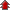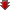# Probability

• NCERT Chapter### Exercise 1

•  Q1 Describe the sample space for the indicated experiment: A coin is tossed three times. Q2 Describe the sample space for the indicated experiment: A die is thrown two times. Q3 Describe the sample space for the indicated experiment: A coin is tossed four times. Q4 Describe the sample space for the indicated experiment: A coin is tossed and a die is thrown. Q5 Describe the sample space for the indicated experiment: A coin is tossed and then a die is rolled only in case a head is shown on the coin. Q6 2 boys and 2 girls are in Room X, and 1 boy and 3 girls in Room Y. Specify the sample space for the experiment in which a room is selected and then a person. Q7 One die of red colour, one of white colour and one of blue colour are placed in a bag. One die is selected at random and rolled, its colour and the number on its uppermost face is noted. Describe the sample space. Q8 An experiment consists of recording boy-girl composition of families with 2 children. (i) What is the sample space if we are interested in knowing whether it is a boy or girl in the order of their births? (ii) What is the sample space if we are interested in the number of girls in the family? Q9 A box contains 1 red and 3 identical white balls. Two balls are drawn at random in succession without replacement. Write the sample space for this experiment. Q10 An experiment consists of tossing a coin and then throwing it second time if a head occurs. If a tail occurs on the first toss, then a die is rolled once. Find the sample space. Q11 Suppose 3 bulbs are selected at random from a lot. Each bulb is tested and classified as defective (D) or non-defective (N). Write the sample space of this experiment? Q12 A coin is tossed. If the out come is a head, a die is thrown. If the die shows up an even number, the die is thrown again. What is the sample space for the experiment? Q13 The numbers 1, 2, 3 and 4 are written separately on four slips of paper. The slips are put in a box and mixed thoroughly. A person draws two slips from the box, one after the other, without replacement. Describe the sample space for the experiment. Q14 An experiment consists of rolling a die and then tossing a coin once if the number on the die is even. If the number on the die is odd, the coin is tossed twice. Write the sample space for this experiment. Q15 A coin is tossed. If it shows a tail, we draw a ball from a box which contains 2 red and 3 black balls. If it shows head, we throw a die. Find the sample space for this experiment. Q16 A die is thrown repeatedly until a six comes up. What is the sample space for this experiment?### Exercise 2

•  Q1 A die is rolled. Let E be the event “die shows 4” and F be the event “die shows even number”. Are E and F mutually exclusive? Q2 A die is thrown. Describe the following events: (i) A: a number less than 7 (ii) B: a number greater than 7 (iii) C: a multiple of 3 (iv) D: a number less than 4 (v) E: an even number greater than 4 (vi) F: a number not less than 3 Also find A ∪ B, A ∩ B, B ∪ C, E ∩ F, D ∩ E, A – C, D – E, E ∩ F’, F’ Q4 Three coins are tossed once. Let A denote the event ‘three heads show”, B denote the event “two heads and one tail show”. C denote the event “three tails show” and D denote the event ‘a head shows on the first coin”. Which events are (i) mutually exclusive? (ii) simple? (iii) compound? Q5 Three coins are tossed. Describe (i) Two events which are mutually exclusive. (ii) Three events which are mutually exclusive and exhaustive. (iii) Two events, which are not mutually exclusive. (iv) Two events which are mutually exclusive but not exhaustive. (v) Three events which are mutually exclusive but not exhaustive.